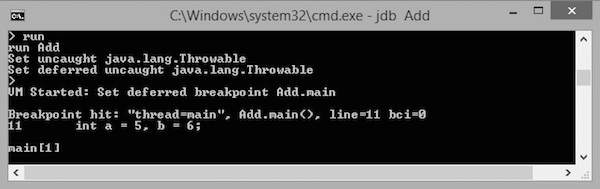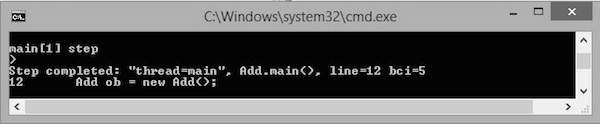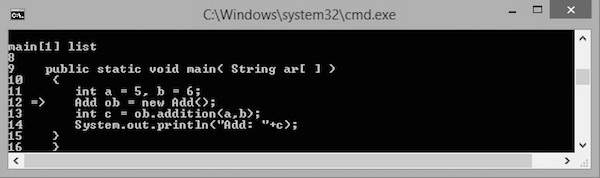# JDB - Stepping

This chapter explains how to use the concept of Stepping in debugging a program. Stepping is the debugger feature that lets you execute the code by stepping through line by line. Using this, you can examine each line of the code to ensure they are behaving as intended.

The following commands are used in the stepping process:

• step: steps to the next line of execution
• list: examines where you are in the code
• cont: continues the remaining execution

## Example

The following example uses the Add class that we have used in the previous chapter:

```public class Add
{
public int addition( int x, int y)
{
int z = x + y;
return z;
}

public static void main( String ar[ ] )
{
int a = 5, b = 6;

}
}
```

Save the above file as Add.java. Compile this file using the following command:

```\>javac Add.java
```

Let us assume that the breakpoint is set on the main() method of the Add class. The following steps show how to apply stepping in the Add class.

## Step 1: Execute the Job

The following command starts executing the class named Add.

```> run Add
```

If you execute this command, you get to see the following output. In this output, you can find that the execution stops at the breakpoint position, i.e., at the main() method.The execution stops at the first line of the main method, that is at "int a=5, b=6;" or Line no: 11 in the code. You can observe this information in the output.

## Step 2: Step through the Code

The following command steps the execution to the next line.

```main step
```

Now the execution steps to Line no: 12. You get to see the following output.## Step 3: List the Code

The following command lists the code:

```main list
```

You get the following output. List command is used to let you know the line in the code up to which the program control has reached. Notice the arrow mark => in the following screenshot that shows the current position of the program control.## Step 4: Continue Execution

The following command continues to execute the code:

```main cont
```

This command continues executing the remaining lines of the code. The output is as shown below:

```> Add:11
The application exited
\>
```

Generally, there are three types of stepping:

• Step Into
• Step Over
• Step Out

## Step Into

Using this command, you can step to the next line of the code. If the next line of the code is a function call, then it enters the function by driving the control at the top line of the function.

In the following code, the arrow mark defines the controller in the code.

```public class Add
{
public int addition( int x, int y)
{
int z = x + y;
return z;
}

public static void main( String ar[ ] )
{
int a = 5, b = 6;

}
}
```

If you use the step into command, the controller moves to the next line, i.e., "int c = ob.addition(a,b);". At this line, there is a function call addition(int, int) hence the controller moves to the topmost line of the addition function with the arrow mark as shown below:

```public class Add
{
public int addition( int x, int y)
-> {
int z = x + y;
return z;
}

public static void main( String ar[ ] )
{
int a = 5, b = 6;

}
}
```

## Step Over

Step Over also executes the next line. But if the next line is a function call, it executes that function in the background and returns the result.

Let us take an example. In the following code, the arrow mark defines the control in the code.

```public class Add
{
public int addition( int x, int y)
{
int z = x + y;
return z;
}
public static void main( String ar[ ] )
{
int a = 5, b = 6;

}
}
```

If you use the step over command, the control moves to the next line, i.e., "int c = ob.addition(a,b);". In this line, there is a function call addition(int, int) hence the function execution is done in the background and the result is returned to the current line with the arrow mark as shown below:

```public class Add
{
public int addition( int x, int y)
{
int z = x + y;
return z;
}

public static void main( String ar[ ] )
{
int a = 5, b = 6;

}
}
```

## Step Out

Step Out executes the next line. If the next line is a function call, it skips that and the function execution continues with the remaining lines of the code.

Let us take an example. In the following code, the arrow mark defines the controller in the code.

```public class Add
{
public int addition( int x, int y)
{
int z = x + y;
return z;
}

public static void main( String ar[ ] )
{
int a = 5, b = 6;

}
}
```

If you use the step out command, the controller moves to the next line, i.e., "int c = ob.addition(a,b);". In this line, there is a function call addition(int, int) hence the function execution is skipped and the remaining execution continues with the arrow mark as shown below:

```public class Add
{
public int addition( int x, int y)
{
int z = x + y;
return z;
}

public static void main( String ar[ ] )
{
int a = 5, b = 6;